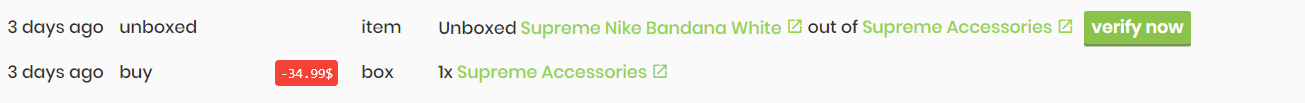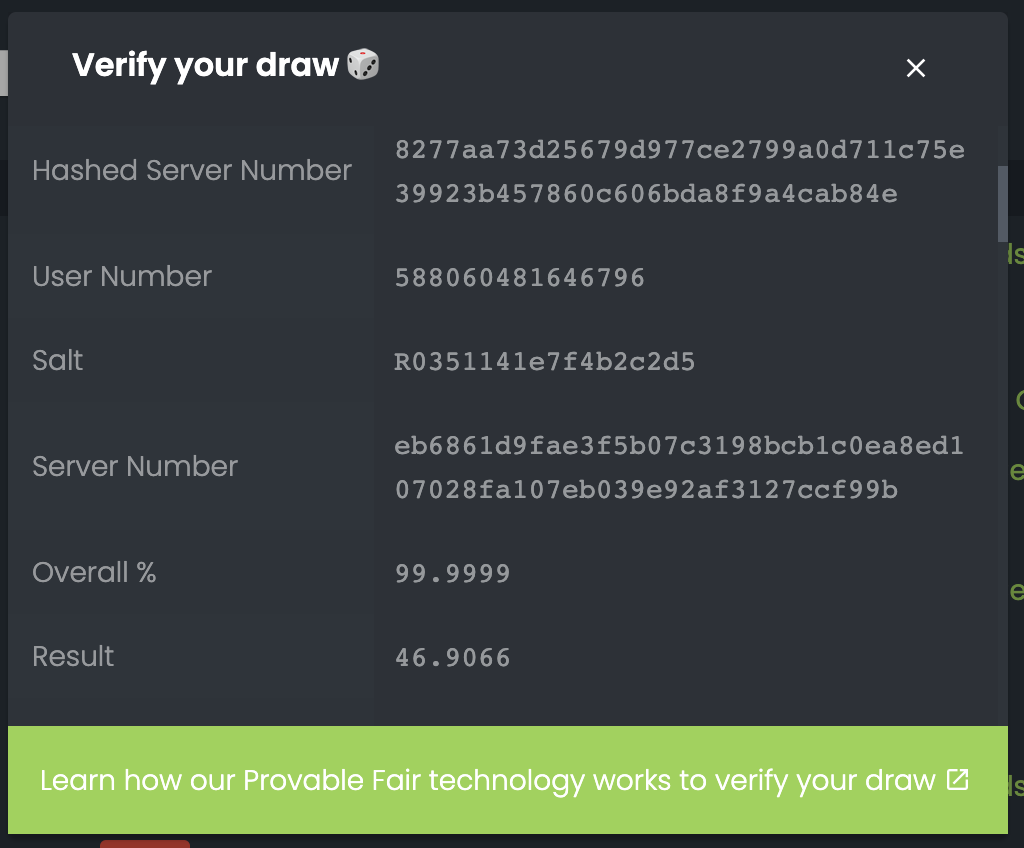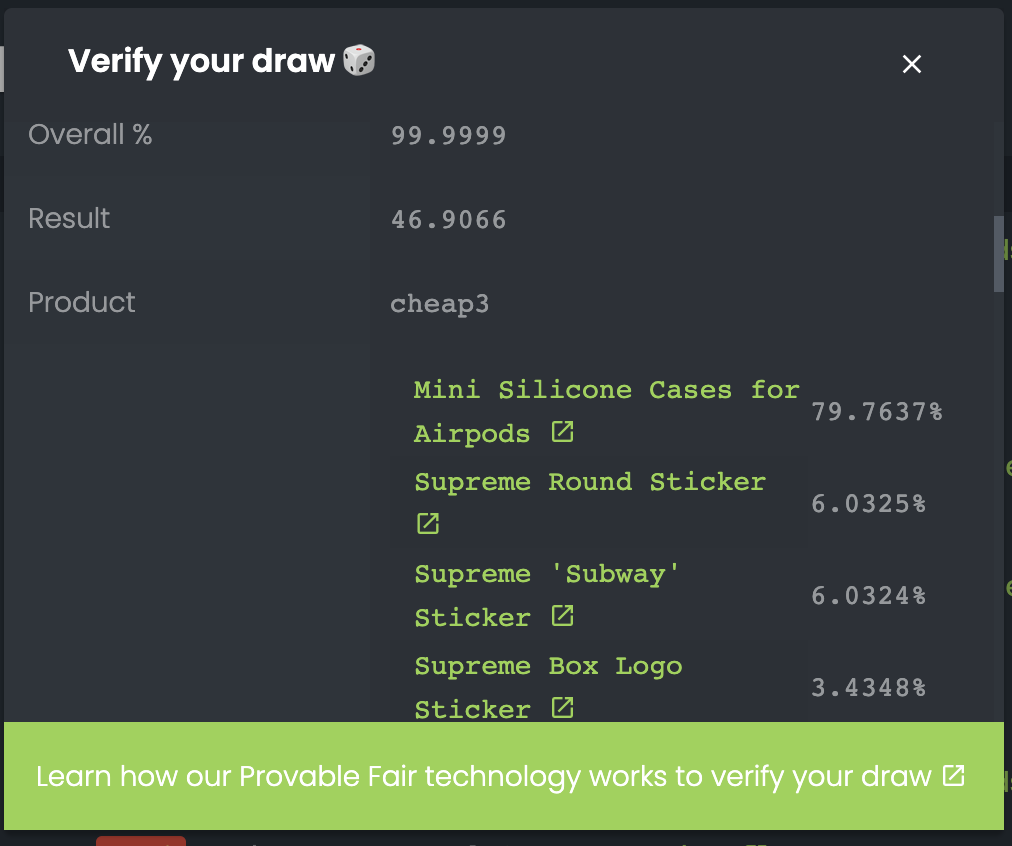# How does "Provable Fairness" work?

All box openings on Dripdraw.com are 100% fair and transparent. Find out how this works and how to verify your draws.Written by Florian
Updated 1 year ago

Transparency and fairness are our top priorities and indeed the reason for the development of Dripdraw. We were scammed by other very reputable competitors in this industry and decided to launch Dripdraw.com. But we do not only claim to be fair and transparent, we can prove it in all drawings.

## What is "Provable Fair" and how does it work?

Fundamentally and broadly speaking, we use the same technology and principle as Bitcoin and other PoW (Proof-of-Work) crypto currencies to ensure that all drawings are fair and provable. You can divide the unboxing procedure into four steps:

1. Before a user can open a box, the server (computer operated by Dripdraw) generates a random number. The number typically consists of 6 to 32 alphanumerical digits. This number is sent to the user and displayed in a SHA256 hashed format. Note that we salt the number first, by applying the salt+number.

However, for general understanding it should be clear to you what a so-called hash algorithm is. More specifically, what the SHA256 hash algorithm is, whereas we can recommend the Wikipedia article to get a basic understanding. In any case, it is important to know that cryptographic hash algorithms guarantee that a hashed input text cannot be converted back again. Once hashed, you have no idea what the original was. It is also important to note that it is very difficult and mathematically almost impossible (like you need a few years with a supercomputer) to calculate the input text for a given hash value.

For example, let's imagine we want to hash "1234" with SHA256:
03ac674216f3e15c761ee1a5e255f067953623c8b388b4459e13f978d7c846f4

The text "1234" will always result in exactly this hash value. At the same time it is mathematically incalculable and impossible to find out from which number this hash value was generated (so we cannot generate a number from the result, unless we already know it).

To improve the security on our side, we add a so called "salt" to the hash. This salt is displayed to you as well before each unboxing. So for example, if the server number is "e886f90344385ae52e20b1b23c4e1e62", and the salt is "0005ec05c3dc8834f26", the hash will be a combination of both: first the salt, and then the server number. In our example, this will result in "0005ec05c3dc8834f26e886f90344385ae52e20b1b23c4e1e62". This number is then SHA256-hashed and sent to the user.

Now we have practically proved that we have already generated a number independent of the user input. Therefore we cannot change the outcome afterwards without breaking the hash.
2. In the second step, the user chooses his own number. This number can be any alphanumerical text he wish to choose. Note that we apply a filter to ensure the number will not contain any harmful content. Hence the requirements for the server and user number are identical.

Once the user has chosen his number, he sends it to the server and thus opens the box.
3. Once the server has received both numbers (its own generated and the user's), it can use a very simple algorithm to calculate a result based on exactly these two parameters. This result is exactly between 0 and 100 and is calculated to an accuracy of precisely 4 decimal places.

The algorithm used to calculate the result:
We use the following algorithm to calculate the roll result, depending on the user and server number.

``````/**
* Calculates the roll result using the user and
* server numbers.
* @param userNumber number/string The chosen number by the user
* @param serverNumber number/string The chosen number by the server
*/
static calculateRollResult(userNumber, serverNumber) {
// Take both numbers and create a string out of it to iterate
// through the digits, required later on.
const combinedNumberStr = userNumber.toString() + serverNumber.toString();
// In order to improve the average result, we need some additional number
// in case the user/server numbers are especially small.
// This also used to strengthen the roll against manipulation.
const strengthFactor = combinedNumberStr.length;

// Now we will iterate through the combined number string
// and modify the sum according to each digit.
let sum = 1;
for (const valChar of combinedNumberStr) {
let val = valChar.charCodeAt();  // Since we support alphanumeric values, we need to convert them into numbers

// We use our parameters to calculate a new sum and keep it below 1.000.000
sum = ((sum * val) * strengthFactor + val + 1) % 1000001 - 1;
}

// Once we are done, we'll have a random number between 0 and 100, with an accuracy to 4 places after the comma.
return parseFloat((sum * 0.0001).toFixed(4));
}``````
We also tested the average result distribution and can guarantee fair outcomes, since it's always very close to 50%:

``````avg. of 100,000 tests: 50.0767
avg. of 100,000 tests: 50.0567
avg. of 100,000 tests: 49.9756
avg. of 100,000 tests: 50.0438
avg. of 100,000 tests: 49.9530``````

To calculate the actual item from this number, the list of all available items sorted by probability is selected, which is within the range of the number.
4. Finally the server sends its number to the user and both, the server and client, can calculate the unboxed item and check if the hash matches the actual server number.

So the result of the draw depends on both: The user and server number. Since we can only influence the server number, we prove with the hash already before opening that we have generated it and that it does not depend on the user number. The user can verify this by checking with a SHA256 online tool whether the hash and the server number match. We can recommend the tool https://emn178.github.io/online-tools/sha256.html

## How to verify the draw?

In principle you can already verify with the server hash that everything went fair, since both numbers (server and client) are needed to calculate the result. To check and verify the result after opening the boxes, we provide a "verify" button on https://dripdraw.com/user?s=1:Using this view, you can check if the roll really went 100% fair and also calculate the product you've drawn.Using this window, you can check the item you have drawn by checking in which range your result number is from the product list. In the example above, our result number was 46.9066%. Since the first (the item with the highest probability) has 79.7637%, it falls into this range.